# Test: Mixtures- 2

## 15 Questions MCQ Test Quantitative Aptitude for GMAT | Test: Mixtures- 2

Description
Attempt Test: Mixtures- 2 | 15 questions in 40 minutes | Mock test for GMAT preparation | Free important questions MCQ to study Quantitative Aptitude for GMAT for GMAT Exam | Download free PDF with solutions
QUESTION: 1

### A solution consists of only water and alcohol such that the ratio of alcohol to water in the solution is 7:3. How much amount of water should be added to the solution (in mililiters) so that the resulting solution contains 60% alcohol? Total quantity of the resulting solution is 350 mililiters. The original solution contains 10.5 mililiters of alcohol for every 4.5 mililiters of water.

Solution:

Step 1 & 2: Understand Question and Draw Inference

Given:

• Ratio of alcohol to water in original solution = 7:3
• Let the quantity of original solution be x ml.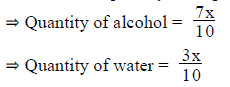To Find:

• We have to find the quantity of water to be added to the solution, such that the resulting solution contains 60% of alcohol.
• Let the quantity of water that is to be added to the solution be y millilitres.
• So total quantity of final solution will be x+y millilitres
• Now since only water is being added we can infer that the quantity of alcohol in the solution will not change.
• So quantity of alcohol in the final solution will be
• Percentage of alcohol in the final solution should be 60%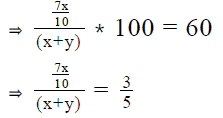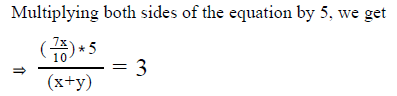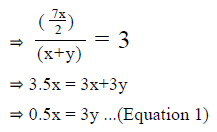• Since we do not know the value of x, we will not be able to determine the value of y.
• So we need a relation in x and y, or value of x or y, to be able to determine the amount of water to be added to the solution.

Step 3 : Analyze Statement 1 independent

• Total quantity of the resulting solution is 350ml.
• Total quantity of the resulting solution = x+y ml (Already established above)

⇒ x+y=350ml ...(Equation 2)

• Since we have another relation in x and y, we will be able to determine the value of y, and thus the amount of water to be added to the solution.
• Hence statement 1 is sufficient to answer the question.

Step 4 : Analyze Statement 2 independent

• The original solution contains 10.5ml of alcohol for every 4.5ml of water.
•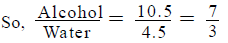• The ratio of alcohol to water from this statement comes out to be 7:3, which is the same as given to us in the question stem.
• So we do not get any additional information from which we can determine the values of x or y.
• Hence statement 2 is not sufficient to answer the question.

Step 5: Analyze Both Statements Together (if needed)

• Since from statement 1, we are able to arrive at a unique answer, combining and analysing statements together is not required.
• Hence the correct answer is option A
QUESTION: 2

### Solution A contains equal amount of alcohol and water in it. It is heated till 50 percent of the water in solution A evaporates. Solution B, whose volume is equal to the reduced volume of water in Solution A, is then added to Solution A and the volume of alcohol in the resultant solution is equal to 12 liters. If solution B contains alcohol and water in the ratio 2:3, how many more liters of water should be added to the resultant solution to increase the concentration of water in the resultant solution to 50 percent?

Solution:

Given:

• Solution A
• Volume of water = x liters (say)
• So, volume of alcohol = x liters (Volume of alcohol and water is equal on the solution)

Reduced volume of water in solution A = 0.5x liters, as 50% of the water evaporates from the original solution having x litres of water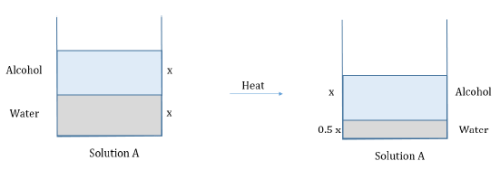• Solution B
• Volume of solution B = 0.5x
• (Volume of solution B is equal to reduced volume of water in solution A, as calculated above)
• Also,(Volume of alcohol): (Volume of water) = 2: 3
• Solution A + Solution B (also called as ‘Resultant Solution’)
• Volume of alcohol = 12 liters

To Find: Amount of water to be added to the resultant solution to increase the concentration of water to 50%?

• Let the amount of water to be added to the resultant solution be y liters.

Approach:

1. We know the volume of water and alchohol in the Solution A in terms of x. We will find the volume of water and alcohol in the Solution B in terms of x, by using the ratio of volume of alcohol to volume of water i.e. 2:3.
2. After knowing the volume of water and volume of alcohol in terms of x in both Solution A and Solution B, we can find the volume of water and alcohol in the resulting solution in terms of x, by adding the constituents of the Solutions A and B.
3. Knowing the volume of alcohol in the resulting solution in terms of x, we will be able to find the value of x, by equating volume of alcohol in resulting solution to 12 liters.
4. After knowing the value of x, we will be able to get the values of volume of alcohol and water in the resulting solution.
5. Now to make the concentration of alcohol to be 50%, we are adding y liters of water. We can finally calculate the value of y, by using the relation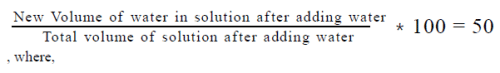• New Volume of water in solution after adding water= Volume of water in resultant solution + y
• Total volume of solution after adding water=Volume of resultant solution+y

Working out: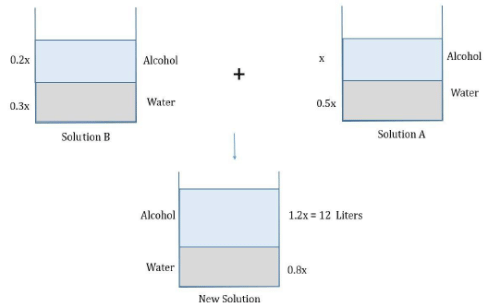1. Volume of solution B = 0.5x
• Volume of alcohol:Volume of water =2:3,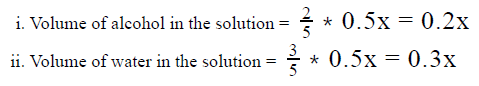2. Volume of Alcohol  in the resultant solution = Volume  of alcohol in solution A + volume of Alcohol in solution B

• Volume of alcohol in resultant solution = x + 0.2x = 1.2x (See  diagram above)
• Now we know that volume of alcohol in the resultant solution = 12  liters
• Thus, 1.2x = 12 , x = 10 liters

3. Volume of Water  in the resultant solution = Volume  of Water  in solution A + volume of Water in solution B

•  Volume of water in resultant solution = 0.5x + 0.3x = 0.8x (See  diagram above)
• So, Volume of water in the resultant solution =0.8*10=8liters (As x=10, already calculated above)

4. Now the amount of water to be added is y liters

• So, New Volume of water in solution after adding water = 8 + y
• Total volume of solution after adding water= 12 + (8+y) = 20 + y
• Now we know the concentration of water is to be made 50%, so,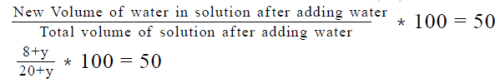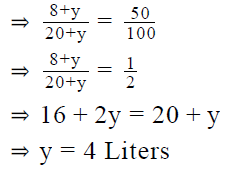5. Hence 4 liters of water needs to be added to increase the volume of water to 50% of the solution

QUESTION: 3

### Solution A is made up of alcohol and water mixed in the ratio of 21:4 by volume; Solution B is made up of alcohol and water mixed in the ratio of 2:3 by volume. If Solution A and Solution B are mixed in the ratio of 5:6 by volume, what percent of the resultant mixture is alcohol?

Solution:

Given: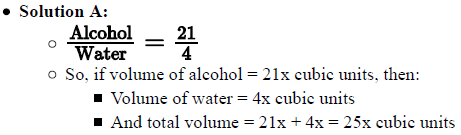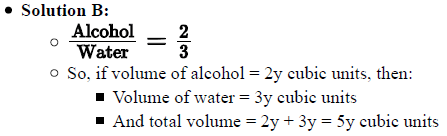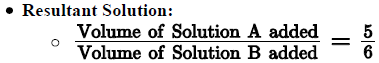To Find: % of alcohol in Resultant Solution

Approach: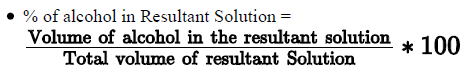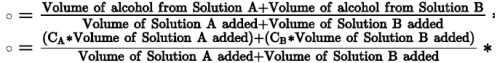• where CA = volume of alcohol in Solution A per unit volume of Solution A and CB = volume of alcohol in Solution B per unit volume of Solution B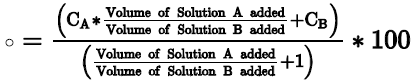We already know the ratio of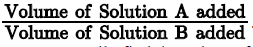And using our inferences in the Given section, we can easily find the values of CB and CB and thus, answer the question.

Working out: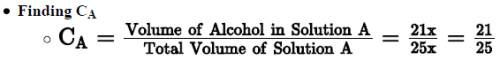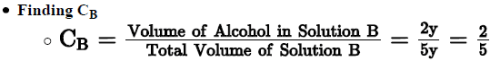• Getting to the required answer
• ​% of alcohol in Resultant Solution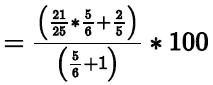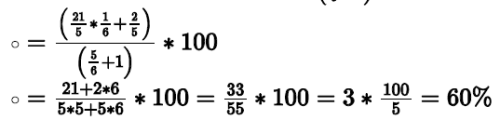Looking at the answer choices, we see that the correct answer is Option C

QUESTION: 4

A 20 kilograms mixture of dry fruits consisting of x percent of almonds is formed by mixing a kilograms of mixture A and b kilograms of mixture B. If mixture A consists of y percent of almonds and mixture B consists of 20% of almonds, what is the weight of almonds in kilograms in mixture A? Assume that each mixture has a uniform composition, that is, samples taken from any part in a mixture will have the same composition as the overall mixture.

(1) The amount of almonds in mixture B is three-fifths of the total weight of mixture A
(2) If the weight of the final mixture is reduced by 25 percent, the almonds in the final mixture would reduce by 1.5  kilograms

Solution:

Step 1 & 2: Understand Question and Draw Inference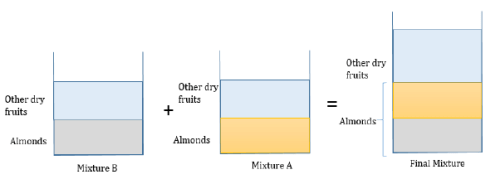• The percentage weight of almond in Mixture A of a kilograms = y%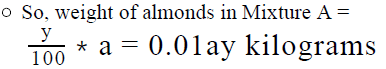• The percentage weight of almond in Mixture B of b kilograms = 20%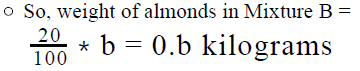• The percentage weight of almond in the final mixture of 20 kilograms is given to be x%
• So, weight of almonds in final mixture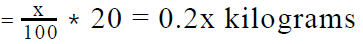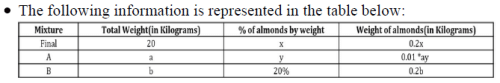• Now, Weight of almonds in the final mixture = Weight of almonds in mixture A + Weight of almonds in mixture B 0.2x = 0.01ay + 0.2b………(1)
• Also, Weight of final mixture = Weight of mixture A + Weight of mixture B
• So a + b = 20……(2)

To Find: We need to find weight of almonds in mixture A

• We know, weight of almonds in mixture A= 0.01*ay
• Thus, we need to  Find  the value of  ay

Step 3 : Analyze Statement 1 independent

• Weight of almonds in mixture B = 0.2b kilograms
• Total weight of mixture A = a kilograms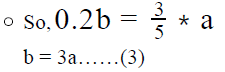• Substituting (3) in (2), we have
• 4a = 20 i.e. a = 5 kilograms and b = 15 kilograms
• Weight of almond in mixture B = 20% of 15 kilograms = 3 kilograms
• However, this does not give us the value of y.

Step 4 : Analyze Statement 2 independent

If the weight of the final mixture is reduced by 25 percent, the almonds in the
final mixture would reduce by 1.5 kilograms

•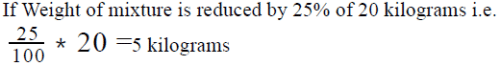• Then, Weight of almonds gets reduced by 1.5 kilograms
• Now since the constituents of mixture has same compostion throughout the mixture
•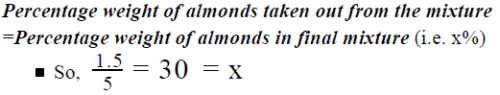• Weight of almonds in final mixture = 30% of 20 kilograms = 6 kilograms
• However, this does not tell us the weight of almonds in mixture A.

Step 5: Analyze Both Statements Together (if needed)

1. Weight of almonds in mixture B = 3 kilograms

a. Weight of mixture A = 5 kilograms

b. Weight of mixture B = 15 kilograms

2. Weight of almonds in final mixture = 6 kilograms

Now, Weight of almonds in final mixture = Weight of almonds in mixture A + Weight of almonds in mixture B

• So, 6 kilograms = weight of almonds in mixture A + 3 kilograms
• Thus,  Weight of Almonds  in  mixture  A =  3 Killogram

QUESTION: 5

A salt-water solution that contains 8% salt by volume is kept in the sun till enough water evaporates from the solution such that the concentration of salt in the solution becomes 20%. If the volume of the remaining solution is 20 liters, what was the volume of the solution before it was kept in the sun?

Solution:

Given:

• Original Solution
• Let the original volume be X Liters
• Salt Volume = 8% of X Liters
• Evaporated Solution
• Volume after Evaporation = 20 liters
• Salt Volume = 20% of 20 = 4 liters

To Find: X = ?

Approach:

1. To find X, we need to draft an equation in X
2. During the process of evaporation, it was the volume of water in the solution that got reduced. The amount of salt was not impacted by the evaporation process. So,
• (Amount of Salt in the original solution) = (Amount of Salt in the Evaporated solution)
• As we know the amount of salt in the evaporated solution, we will use this equation to find the value of X

Working out:

• Amount of Salt in the original solution = 8x/100  liters
• As inferred above, Amount of Salt in the final solution = 4 liters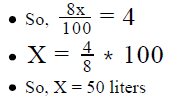Looking at the answer choices, we see that the correct answer is option D

QUESTION: 6

A container consists of x liters of milk. The milk in the container is adulterated by adding water equal to 50 percent of the milk in
the container. If a person takes out 6 liters of the milk solution from the container, how many liters of water does he take out?

Solution:

Given:

• Volume of milk in the container = x liters
• Volume of water added in the container= 0.5x liters
• Amount of milk solution taken out = 6 liters

To Find: The amount of water taken out from the milk solution?

Approach: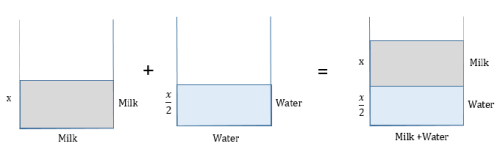1. We will find the volume of water taken out from the solution by calculating the total percentage of water in the milk solution.
• From the volume of individual constituents, we will find the total volume of milk solution in terms of x.
• We know the volume of individual constituents in the milk solution in terms of x.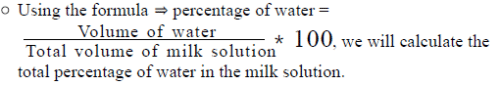2. Using the same formula as above and putting the absolute value of total volume of milk solution (6 liters) and the calculated percentage, we will be ble to find the water taken out from the solution.

Working out:

1. Volume of milk = x liters

2. Volume of water = 0.5x liters
3. Total volume of milk solution = x + 0.5x = 1.5x liters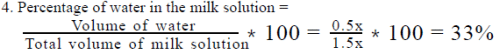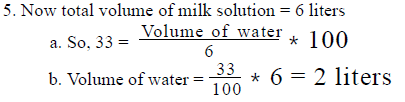6. Hence, amount of water in 6 liters of milk solution is 2 liters

QUESTION: 7

A solution contains ethanol, methanol and water in the ratio of 1:4:15 by volume. The composition of this solution is altered by adding ethanol, methanol and water such that the amount of ethanol per unit volume of methanol increases by 15 percentage points and the amount of water per unit volume of ethanol decreases by two-thirds. If the volume of the altered solution is 340 cubic units, which of the following is the volume, in cubic units, of methanol in the altered solution?

Solution:

Given:

• Original Solution:
• Let Amount of Ethanol = x cubic units
• So, Amount of Methanol = 4x cubic units
• And, Amount of Water = 15x cubic units
• In the original solution,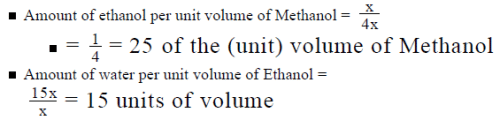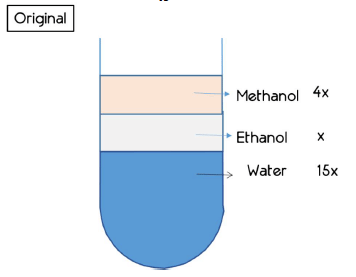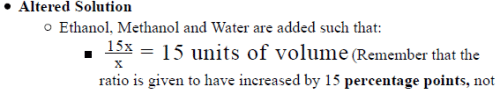by 15 percent)

• So, New volume of Ethanol = 40% of (New volume of Methanol)
• New volume of Ethanol = 0.4(New Volume of Methanol)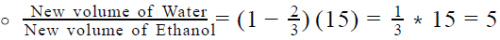• So, New volume of Water = 5(New Volume of Ethanol)
• Let the New volume of Methanol be y cubic units
• So, the new volume of Ethanol = 0.4y
• And, the new volume of water = 5*0.4y = 2y
• Total volume of the altered solution = 340 cubic units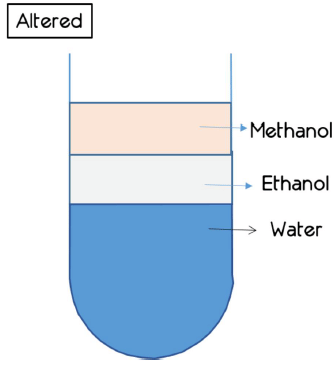To Find : y = ?

Approach:

1.  To find y, we need an equation in y. This equation is easily obtained by equating the known total volume of the Altered Solution to the sum of the volumes of Ethanol, Methanol and Water in the Altered Solution
• (Volume of Methanol in the Altered Solution) + (Volume of Ethanol in the Altered Solution) + (Volume of Water in the Altered Solution) = (Total Volume of the Altered Solution)

Working out:

• Using the equation written in Approach, we get: y + 0.4y + 2y = 340
• So, 3.4y = 340
• That is, y = 100 cubic units

Looking at the answer choices, we see that the correct answer is Option D

QUESTION: 8

Packet A contains a mixture of salt and sugar and Packet B contains a mixture of salt and pepper. When the contents of the two packets are mixed in a bowl, the resultant mixture weighs 400 grams and contains 25% sugar. How many grams of salt are contained in Packet B?

(1) One-third of the mixture in Packet A is sugar
(2) 15% of the resultant mixture is pepper

Solution:

Step 1 & 2: Understand Question and Draw Inference

Given:
Let packet A contain x grams of Salt and y grams of Sugar
Let packet B contain z grams of Salt and w grams of pepper
So, we can represent the 2 packets A and B visually as below: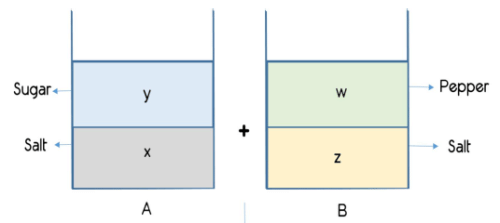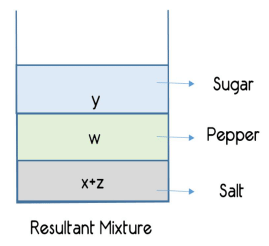• The total weight of the resultant mixture = x + y + z + w = 400 grams. . . (I)
• y = 25% of 400 = 100 grams . . . (II)

To Find: the value of z

Step 3 : Analyze Statement 1 independent

Statement 1 says that One-third of the mixture in Packet A is sugar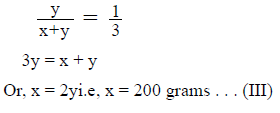By substituting (III) and (II) in (I), we get a linear equation with 2 unknowns: z and w.
Not sufficient to find a unique value of z.

Step 4 : Analyze Statement 2 independent

Statement 2 says that 15% of the resultant mixture is pepper
w = 15% of 400 = 60 grams . . . (IV)
Substituting (II) and (IV) in (I) gives us a linear equation with 2 unknowns: x and z.
Not sufficient to find a unique value of z.

Step 5: Analyze Both Statements Together (if needed)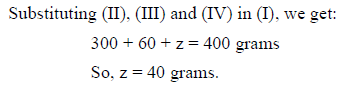Sufficient

QUESTION: 9

A juice is made by mixing x liters of mango pulp with y liters of orange pulp and 14 liters of water where x and y are non-zero integers, mango pulp costs \$6 per 10 liters and orange pulp costs \$4 per 10 liters, is x >3?

(1) If water is available free of charge, the juice costs \$1.6 per 10 liters
(2) If mango pulp had cost 20 cents more per liter, then the cost of the mango pulp used in the juice would have been more than \$2 greater than the cost of the orange pulp used in the juice

Solution:

Step 1 & 2: Understand Question and Draw Inference

Given:

• Volume of:
• Mango pulp = x liters, where x is a non-zero integer
• Orange pulp = y liters, where y is a non-zero integer
• Water = 14 Liters
• Total volume of juice = x + y + 14 liters
• Cost per liter of:
• Mango pulp = \$0.6
• Orange pulp = \$0.4
• Water is unknown

To find: Is x > 3?

Step 3 : Analyze Statement 1 independent

• Statement 1 says that ‘If water is available free of charge, the juice costs \$1.6 per 10 liters’
• Cost per liter of:
• Water = \$0
• Cost of 10 liters of juice = \$1.6
• So, cost per liter of juice = \$0.16
• (Volume of Mango pulp per liter of juice)*(Cost per liter of Mango pulp) + (Volume of Orange pulp per liter of juice)*(Cost per liter of Orange pulp) +(Volume of water) * (Cost per liter of Water) = \$0.16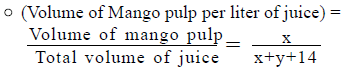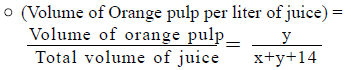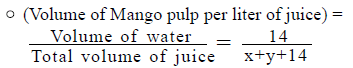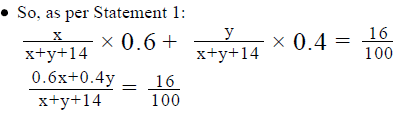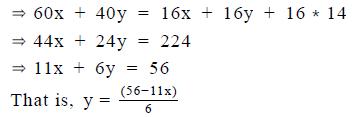• Constraints on x and y:

• Both are non-zero integers
• Since both represent volumes, they cannot be negative.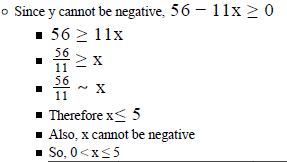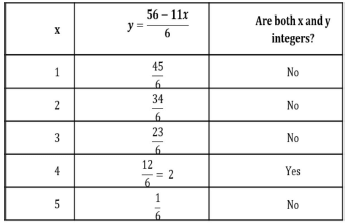• Only possible value of x is 4
• Since we now know a unique value of x, we can answer the question. Suffecient

Step 4 : Analyze Statement 2 independent

Statement 2 says that ‘If mango pulp had cost 20 cents more per liter, then the cost of the mango pulp used in the juice would have been more than \$2 greater than the cost of the orange pulp used in the juice’

• As the original cost per liter of mango pulp was \$0.6,
• New Cost per liter of:
• Mango pulp =\$0.6 + 20 cents = \$0.6 + \$0.2 = \$0.8
• So, price of x liters of mango pulp = \$0.8x
• Also, as the price per liter of orange pulp is given to be \$0.4, priceof y liters of orange pulp = \$0.4y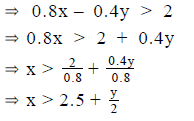• Constraints on x and y:
• Both are non-zero integers
• The minimum value of x will happen when the value of y is minimum
• Since both represent volumes, they cannot be negative.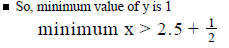• Thus, minimum value of x is greater than 3.

Sufficient.

Step 5: Analyze Both Statements Together (if needed)

Since we get a unique answer in each of Steps 3 and 4, this step is not required.

QUESTION: 10

A tea company sells two brands of tea leaves, Brand A and Brand B. Both the brands of tea leaves consist of three types of tea leaves, types-I, II and III, but in different weights. The cost of a kilogram of a brand of tea leaves is given by the expression \$(100p+80q+50r), where p, q and r are the weights of type-I, II and III tea leaves respectively in 1 kilogram of that brand of tea leaves. Which of the two brands of tea leaves costs more?

(1) The individual percentage weights of type-I and type-III tea leaves are greater in Brand A than in Brand B
(2) The percentage weight of type-I tea leaves in Brands A and B is 40 percent and 20 percent respectively

Solution:

Step 1 & 2: Understand Question and Draw Inference

Given:

• Two brands of Tea – A and B
• Both contain leaves of Type – I, II, and III
• Cost of 1 kg of Tea = 100p + 80q + 50r
• p = weight of leaves of Type I
• q = weight of leaves of Type II
• r = weight of leaves of Type III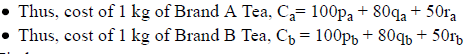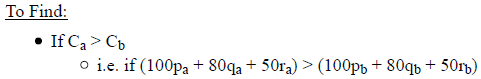Step 3 : Analyze Statement 1 independent

The individual percentage weights of type-I and type-III tea leaves are greater
in Brand A than in Brand B.

• Information given about % weight of leaves of Type I & Type III
• Note: % information can be directly used for weights of components
• Since, we are dealing with 1 kg of Tea of each brand
• Thus,
pa > pb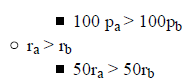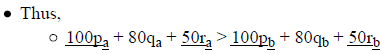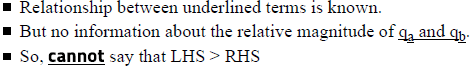• Thus, the information in this statement is not sufficient to answer the question.

Step 4 : Analyze Statement 2 independent

The percentage weight of type-I tea leaves in Brands A and B is 40 percent and
20 percent respectively
Information given about % weight of leaves of Type I
Note: Since, we are dealing with 1 kg of Tea of each brand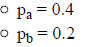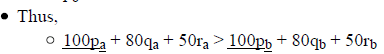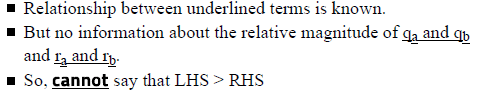• Thus, the information in this statement is not sufficient to answer the question.

Step 5: Analyze Both Statements Together (if needed)

1. From Statement 1, we had no information about the relative magnitude of qa and qb
2. From statement 2, we had no information about the relative magnitude of qa and qb and ra and rb .
3. Even by combining the statements no extra information about the relative magnitude of qa and qb will be available.
4. So by combining both the statements, we will not be able to sufficiently answer the question.

QUESTION: 11

A solution contains water, milk and liquid chocolate in the ratio of 2:3:5 by volume. If y liters of water and milk each are added to this solution, the resultant solution would contain 25 percent of water by volume. If the volume of this resultant solution is 120 liters, what is the value of y in liters?

Solution:

Given:

• Ratio of water, milk and liquid chocolate in the original solution is 2:3:5
• Let the volume of water, milk and liquid chocolate in the original solution be 2x, 3x and 5x respectively
• Total volume of the original solution= 2x+3x+5x = = 10x
• Water added to the original solution= y liters
• Milk added to the original solution= y liters
• Total volume of the resultant solution = 120 liters
• Volume of water in the resultant solution = 25% of the total volume
• So, Volume of water in the resultant solution= 25% of 120 = 30 liters

To Find: Value of y?

Approach :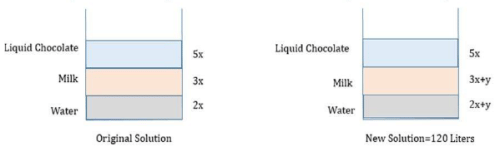1. We will first find the volume of water in the resultant solution after addition of water to the original solution. This will give us one equation in x and y.

a. Volume of water in the original solution =2x liters
b. Volume of water added to the original solution =y liters
c. So, Volume of water in the resultant solution = (2x+y) liters
d. The total volume of water in the new solution is also equal to 30 liters.

• So,we have,2x + y = 30 (Equation 1).

2. We will then find the total volume of the resultant solution to get another equation in x and y.

• Volume of water in the resultant solution = 2x+y (Already established above)
• Volume of milk in the resultant solution = 3x+y (3x liters milk in original solution added to y liters of milk solution)
• Volume of liquid chocolate in the resultant solution =5x liters (No liquid chocolate is added)
• So, total volume of the resultant solution = (2x+y)+(3x+y)+(5x) =10x+2y liters
• The total volume of solution is also equal to 120 liters.
• So, we have, 10x+2y=120 (Equation 2)

3. Using the two equations in x nd y, we will be able to find the value of y as asked in the question.

Working out:

1. From equation 1, we have 2x + y = 30
2. From equation 2, we have 10x+2y=120
3. Solving equation 1 and 2
• Performing (Equation 1) *5, we get 10x+5y =150 (Equation 3)
• Doing (Equation 3) – (Equation 2), we get
• (10x+5y)-(10x+2y)=150-120
• 3y=30
• y=10 liters

4. So water to be added to the original solution is 10 liters.
5. So answer option B is correct.

QUESTION: 12

A vessel is filled with liquid, 3 parts of which are water and 5 parts of syrup. How much of the mixture must be drawn off and replaced with water so that the mixture may be half water and half syrup?

Solution:

Let the quantity of the initial liquid in the vessel = 8 litre and
quantity of water in the initial liquid = 3 litre and
quantity of syrup in the initial liquid = 5 litre
Let x litre of the mixture is drawn off and replaced with waterQuantity of water in the new mixture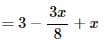Quantity of syrup in the new mixture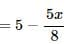Given that in the new mixture, quantity of water = quantity of syrup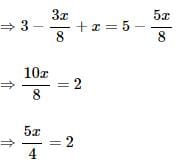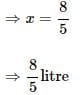Initially we assumed that the quantity of the initial liquid in the vessel = 8 litre for the ease of calculations. For that 8/5 litre of the mixture to be drawn off and replaced  with water so that the mixture may be half water and half syrup
Now, if the initial liquid in the vessel = 1 litre, quantity of the mixture to be drawn off  and replaced with water so that the mixture may be half water and half syrup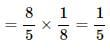It means 1/5 of the mixture has to be drawn off and replaced with water so that the mixture

may be half water and half syrup

QUESTION: 13

A merchant has 1000 kg of sugar part of which he sells at 8% profit and the rest at 18% profit. He gains 14% on the whole. The Quantity sold at 18% profit is

Solution:

By the rule of alligation,we have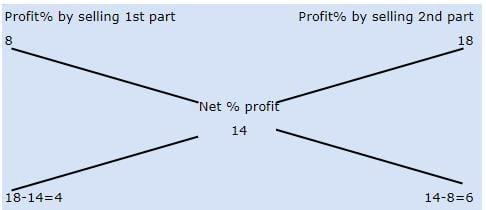=> Quantity of part1 : Quantity of part2 = 4 : 6 = 2 : 3

Total quantity is given as 1000Kg
So Quantity of part2 (Quantity sold at 18% profit)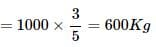QUESTION: 14

John bought 20 kg of wheat at the rate of Rs.8.50 per kg and 35 kg at the rate of Rs.8.75 per kg. He mixed the two. Approximately at what price per kg should he sell the mixture to make 40% profit as the cost price?

Solution: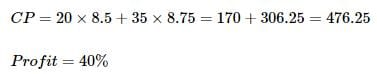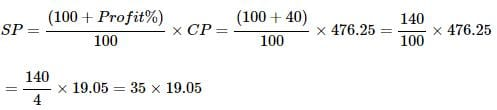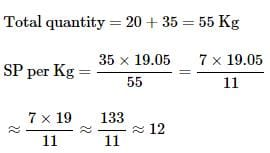QUESTION: 15

A butler stole wine from a butt of sherry which contained 32% of spirit and then replaced what he stole by wine containing only 18% spirit. The butt was then of 24% strength only. How much of the butt had he stolen?

Solution: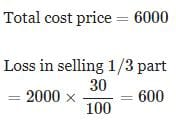So, profit amount by selling remaining
2/3 part =600
cost price of 2/3 part =4000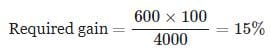Use Code STAYHOME200 and get INR 200 additional OFF Use Coupon Code